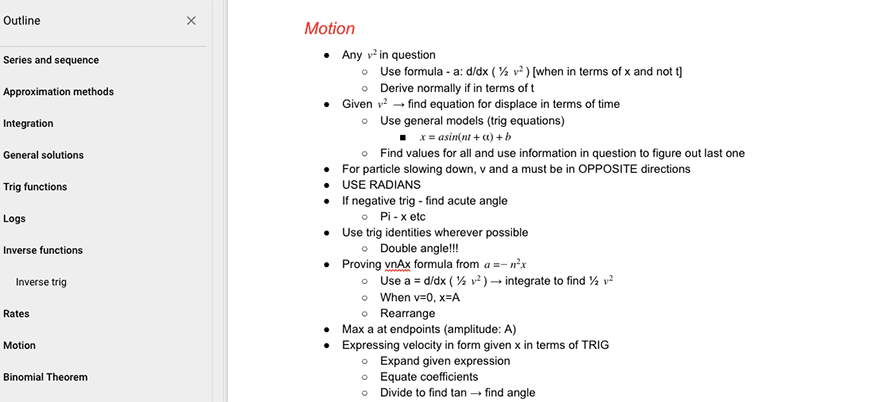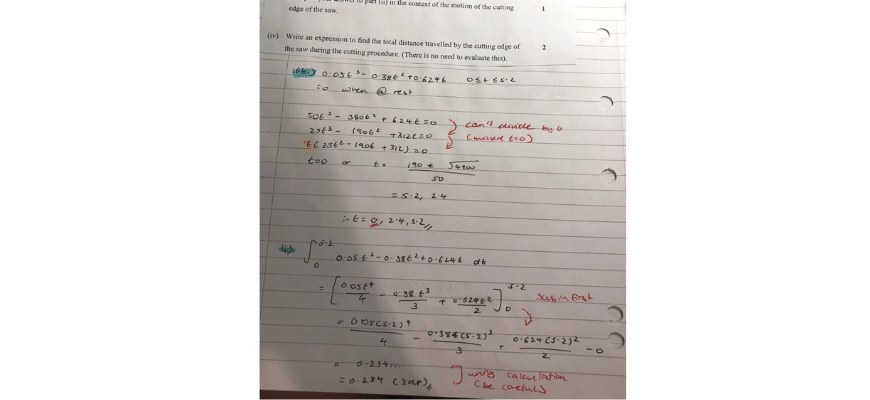# Beginner’s Guide to Year 12 Maths Extension 2 | Overview

Studying Maths Extension 2 doesn't have to be hard! In our Beginner's Guide, we will break down each topic to help you ace your HSC.Are you struggling with Maths Extension 2 Concepts? Well, don’t worry! In our Beginner’s Guide to Year 12 Maths Extension 2, we provide a detailed introduction with practice questions and worksheets to help you get on top of your study.

## All about the Beginner’s Guide to Year 12 Maths Extension 2

Now that you are in Year 12, it is essential that students build on their foundational knowledge from Years 7-11 and grow that knowledge.

This Guide goes through Year 12 Maths Extension 2 HSC content and skills that you need to master!

We break down each concept into clear, concise and manageable chunks of theory, and provide you with some practice questions.

Our purpose for writing this guide is to create clarity around the Year 12 Maths Extension 2 Syllabus.

## What’s in this guide?

Note: Some Maths Ext 2 topics are covered in the Maths Ext 1 Guides.

## How will this Guide help me?

This Guide will provide clarity over the syllabus topics and introduce to the concepts required for study!

We break down the topics and provide challenging questions at the end of each article to help you prepare for your HSC.

We also examine concepts behind different questions, instead of examining pure calculations. This is because we believe that it is beneficial for you to understand Maths, as opposed to simply listing steps to the right answer.

This is our philosophy behind our Matrix Maths Method.

## What are some common student learning difficulties?

In Year 12 Maths Extension 2, many students encounter these problems:

• Students are afraid to take the leap of faith! If students lack the experience and confidence with fundamental principles of number theory, they will be unable to make the required assumptions to solve or prove the problem they’ve been presented with.

• Inability to link concepts together. If students lack conceptual understanding of the topics in Maths Ext 1, they will struggle to tackle questions which rely on multiple concepts.

For example:

Let $$α,β,γ$$ be the roots of $$p(x)=x^3+px+q$$ where $$p,q∈R$$ and $$q≠0$$.

1. Show that $$(β-γ)^2=α^2+\frac{4q}{α}$$
2. Show that $$(α-β)^2 (α-γ)^2 (β-γ)^2=\frac{-1}{q} (3q-pγ)(3q-pβ)(3q-pα)$$
3. Deduce that $$(α-β)^2 (α-γ)^2 (β-γ)^2=-(27q^2+4p^3)$$
4. Hence prove that if $$27q^2+4q^3<0$$, then $$p(z)$$ has three distinct real roots.

• Struggle to communicate the topics properly. When students rote learn maths, they lack a strong conceptual understanding of the topics. This often causes them to struggle with communicating topics.
• Unable to do difficult questions. Many students come across a difficult question and give up without trying it. This is detrimental as it impedes their confidence with answering more challenging questions in the future.

## What do E4 students do differently?

So, now that we know what the most common Maths issues are, let’s examine how you can overcome these issues!

• Have a strong algebraic understanding. Don’t simply push through your Year 11 and 12 contents. Instead, Band 6 students have an awareness of their weaknesses, and consistently go back to topics from previous years and practise algebraic skills.

• Keep a logbook of difficult questions. Many students do practice questions to improve. However, when they reach a difficult question, they simply skip it! This is ineffective, because you are not expanding your skillset.  So, instead, you should keep a logbook of all the difficult questions and allocate some time to figure out the process.Image: Jessy’s logbook of difficult questions

• Consistently give yourself feedback. How can you improve if you don’t mark your own work and figure where you went wrong? So, you should keep a logbook of all the questions you answered incorrectly, then write the correct solution. You should revisit these questions before the exam to refresh your skills.• Be inquisitive. You should always ask questions if you don’t understand something, or want clarification! Remember, if you have a stronger understanding of a Mathematical concept, it will be easier to solve the questions.

• Be disciplined. Don’t just study for Maths the day before your exams! That’s not enough time. Maths is a subject that you need to consistently learn and practice. So, set some time to understand and break down a concept. Then make it your mission to continue doing practice questions to reinforce your knowledge.

• Communicate what you understand. The best way to learn is to teach someone else! So, explain your Maths concepts to your friends, and guide them through the steps of different Maths questions. This will help you remember and understand the concepts better, and it will also reveal areas that you don’t completely understand.

## Last chance to boost your marks in the final HSC exam

Our HSC experts will revise and break down Maths concepts through our structured video lessons, and our mock exam will test your strengths and weaknesses. Learn more about our HSC Exam Prep Course now.© Matrix Education and www.matrix.edu.au, 2021. Unauthorised use and/or duplication of this material without express and written permission from this site’s author and/or owner is strictly prohibited. Excerpts and links may be used, provided that full and clear credit is given to Matrix Education and www.matrix.edu.au with appropriate and specific direction to the original content.